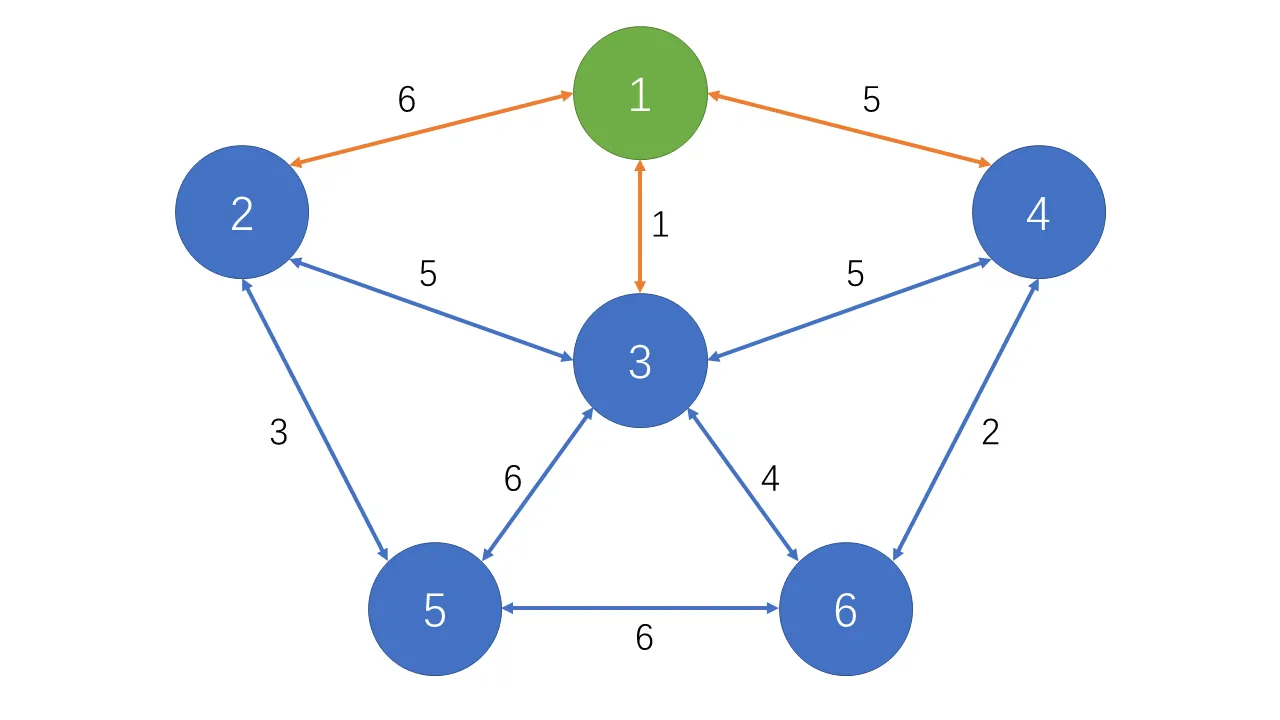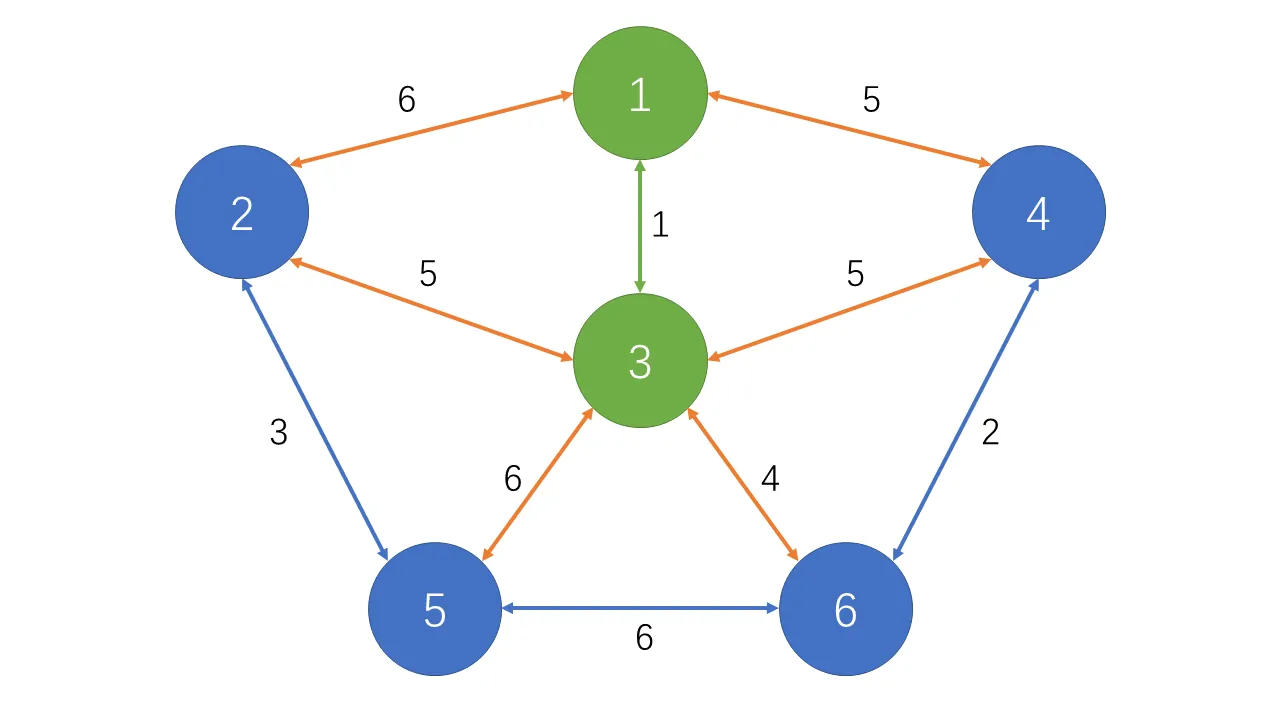# Prim 算法

## 朴素版本

### 复杂度

$\left|V\right|$ 为顶点数，该版本适合稠密图，即 $\left|E\right|接近\left|V\right|^2$ 的图。

### 分析prim (G, dist[])
初始化: dist[1~v]=+INF, dist=0, ans=0
for (循环v次)
t=未选择的点的集合中dist[t]最小的顶点
if (t为无穷大)
return 图不连通
ans加上dist[t]
记t已选择
for (j : 从t出发能到达的顶点的集合)
if (若t到j的距离小于j到已选择点的距离)
更新dist[j];
return ans

### 代码实现

const int MAXN = 510, INF = 0x3f3f3f3f; // INF代表无穷大
int g[MAXN][MAXN]; // 邻接矩阵存图
int dist[MAXN];    // 距离已选择点的最短距离
int vis[MAXN];     // 点是否选择
int v, e;          // v顶点数 e边数

int prim()
{
int ans = 0;
memset(dist, 0x3f, sizeof(dist));
dist = 0;
for (int i = 0; i < v; i++)
{
int t = -1;
for (int j = 1; j <= v; j++)
if (!vis[j] && (t == -1 || dist[t] > dist[j]))
t = j;
if (dist[t] == INF)
return INF;
ans += dist[t];
vis[t] = true;
for (int j = 1; j <= v; j++)
dist[j] = min(dist[j], g[t][j]);
}
return ans;
}

## 堆优化版本

### 复杂度

$\left|E\right|$ 为边数，$\left|V\right|$ 为顶点数，该版本适合稀疏图，即 $\left|E\right|\ll\left|V\right|^2$ 的图。

### 代码实现

typedef pair<int, int> PII;
const int MAXN = 100010, INF = 0x3f3f3f3f;           // INF代表无穷大
int E, V;                                            // E边数 V顶点数
vector<PII> edge[MAXN];                              // 储存边，二元组为(权值,终点)
priority_queue<PII, vector<PII>, greater<PII>> pque; // 短的边在堆顶
int dist[MAXN];                                      // 储存节点到已选择点的最短距离
bool vis[MAXN];                                      // 是否已选择该点

int prim()
{
int ans = 0, cnt = 0;
memset(dist, 0x3f, sizeof(dist));
dist = 0;
pque.push({dist, 1});
while (!pque.empty())
{
PII cur = pque.top();
pque.pop();
if (vis[cur.second])
continue;
ans += cur.first;
cnt++;
vis[cur.second] = true;
for (auto next : edge[cur.second])
{
if (dist[next.second] > next.first)
{
dist[next.second] = next.first;
if (!vis[next.second])
pque.push({dist[next.second], next.second});
}
}
}
return cnt == V ? ans : INF;
}